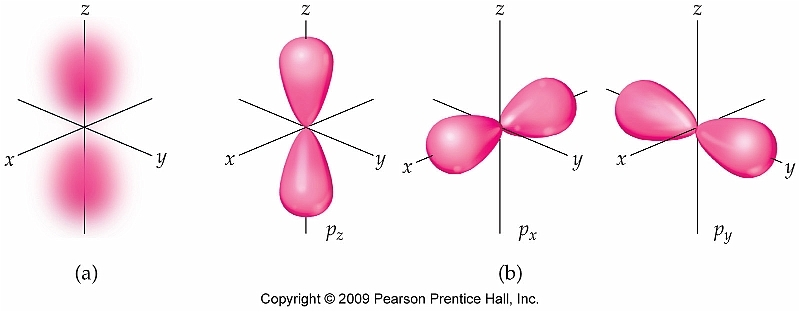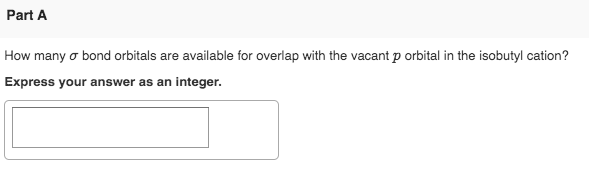# How many orbitals in a p sublevel?

How many orbitals in a p sublevel?

Answer 1

Three.

#### Explanation:

The number of orbitals you get per sublevel is given by the magnetic quantum number, ${m}_{l}$.

More specifically, the number of orbitals you get for a given sublevel depends on the number of values that ${m}_{l}$ can take.

The magnetic quantum number depends on the value of the angular momentum quntum number, $l$, which in turn depends on the principal quantum number, $n$.

SImply put, the principal quantum number gives the energy level on which the electron resides, the angular momentum quantum number gives you the sublevel, and the number of values you have for the magnetic quantum number gives you the number of orbitals you get per sublevel.

So, in order to have a p-sublevel, you need to have $n > 1$, since the first energy level only has an s-sublevel.

The angular momentum quantum number can take values in the range

$l = 0 , 1 , 2 , n - 1$

The magnetic quantum number can take values in the range

${m}_{l} \in \left\{- l , \ldots , 1 , 0 , 1 , \ldots , l\right\}$

A p-sublevel is characterized by $l = 1$, which means that ${m}_{l}$ can be

${m}_{l} = \left\{- 1 , 0 , 1\right\}$

Therefore, the p-sublevel has a total of three p-orbitals: ${p}_{x}$, ${p}_{y}$, and ${p}_{z}$.#### Earn Coin

Coins can be redeemed for fabulous gifts.

Similar Homework Help Questions

• ### Part A How many o bond orbitals are available for overlap with the vacant p orbital...Part A How many o bond orbitals are available for overlap with the vacant p orbital in the isobutyl cation? Express your answer as an integer. Part B How many o bond orbitals are available for overlap with the vacant p orbital in the n-butyl cation? Express your answer as an integer. Part C How many o bond orbitals are available for overlap with the vacant p orbital in the sec-butyl cation? Express your answer as an integer.